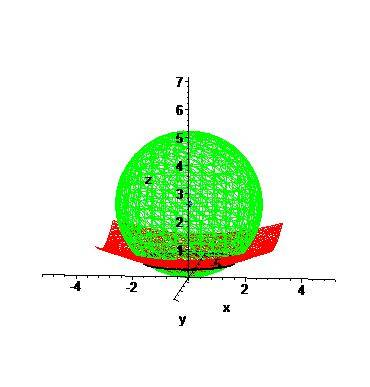# Triple integral - Volume of a sphere and surface

MarcMTL

## Homework Statement

Find the volume of the solid which is contained by 1) $$z= \frac{\sqrt{2}}{4}\sqrt{x^2+y^2}$$ and 2) $$x^2+y^2+z^2= \sqrt{27}z$$

## Homework Equations

I've completed the square on the 2nd equation to obtain $$x^2+y^2= 8z^2$$

and also the 1st equation to obtain $$x^2+y^2+(x-\frac{1}{2}\sqrt{27})^2=\sqrt{\frac{27}{4}}$$

## The Attempt at a Solution

So we're basically looking for a sphere which is cut off at the bottom by the 1st equation. I though it'd be good to solve using spherical coordinates. For the $$\rho$$, i've obtained $$\rho^2 = \sqrt{27}z$$ and $$\rho = 27cos(\phi)$$

$$\theta$$ from 0 to 2$$\pi$$

But I can't for the life of me figure out how to find $$\phi$$? I figure it wont go from 0 to $$\pi$$ because of the bottom limit.

As well, is using spherical the best solution in this case?

Thanks,

Marc

Last edited:

Homework Helper
Gold Member
$$x^2+y^2+(x-\frac{1}{2}\sqrt{27})^2=\sqrt{\frac{27}{4}}$$

<snip>

As well, is using spherical the best solution in this case?

Thanks,

Marc

The sphere should have 27/4 on the right side, without the square root.

From your statement of the problem, which volume "enclosed" by the surfaces isn't clear. I assume it is the volume above your second surface and below the sphere. To answer your question, yes, spherical coordinates is the best way to attack this problem.

Do you realize the second [lower] surface is a cone? The central angle of that cone is going to tell you the $\phi$ limits. Do you understand what the $\rho$ limits will be?

MarcMTL
LCKurtz,

Yes, you're correct, it's the volume located above the cone and below the sphere.

As for the $$\phi$$, how do I go abouts to finding the angle, or more specifically, the central angle of the cone? I know I can figure out the intersect between the both solids, but can't seem to deduce the angle from there. As for the $$\rho$$, I can't seem to calculate it for the cone.

Using $$x^2+y^2=8z^2$$, in spherical I get $$\rho^2(sin(\phi))^2=8(cos(\phi))\rho^2$$, thus meaning the $$\rho$$ cancels out. Does that mean my limits will be from 0 to $$\sqrt{27}cos(\phi)$$ (the $$\rho$$ of the sphere)?

EDIT: What seems to be confusing me is that the cone doesn't intersect the sphere at its half, where we'd have a cone on the bottom supporting the upper half of the sphere. The shapes intersect near the bottom of the sphere.

Thanks for the help.

Marc

Last edited:
Homework Helper
Gold Member
LCKurtz,

Yes, you're correct, it's the volume located above the cone and below the sphere.

As for the $$\phi$$, how do I go abouts to finding the angle, or more specifically, the central angle of the cone? I know I can figure out the intersect between the both solids, but can't seem to deduce the angle from there.

The traces of the cone in the xz or yz planes are straight lines. For example, if x = 0 you get

$$z= \frac{\sqrt{2}}{4}\sqrt{y^2}= \frac{\sqrt{2}}{4}\ |y|}$$

Use the slope of those lines to get the central angle.
As for the $$\rho$$, I can't seem to calculate it for the cone.

Using $$x^2+y^2=8z^2$$, in spherical I get $$\rho^2(sin(\phi))^2=8(cos(\phi))\rho^2$$, thus meaning the $$\rho$$ cancels out. Does that mean my limits will be from 0 to $$\sqrt{27}cos(\phi)$$ (the $$\rho$$ of the sphere)?

Yes, that's exactly what it means. One way to visualize it is to think of a point somewhere inside the volume. Think about moving that point in the $\rho$ direction. It will go from the origin to the sphere, never hitting the cone.

The reason that you can't figure out $\rho$ on the cone is that the equation of the cone is independent of $\rho$. Put the cone in spherical coordinates and you will see.

Homework Helper
Gold Member
EDIT: What seems to be confusing me is that the cone doesn't intersect the sphere at its half, where we'd have a cone on the bottom supporting the upper half of the sphere. The shapes intersect near the bottom of the sphere.

That sphere isn't centered at the origin you know. How do you have it pictured?

MarcMTL
This is my maple graph of the problem:Homework Helper
Gold Member
Your picture looks OK (a parametric plot would look nicer). $\rho$ still goes from 0 to the equation of the sphere.

I should have also mentioned that from your equation (with the cosine squared as it should be):

$$\rho^2(sin(\phi))^2=8(cos^2(\phi))\rho^2$$

that you get

$$\tan^2(\phi) = 8$$

from which you can also get the central angle.

MarcMTL
Thank you very much Lynn. I believe I've grasped the concept here. I'll do a couple more to make sure it sinks in :).

For my limits I obtain:

$$\theta$$ 0 to 2Pi
$$\phi$$ 0 to arccos(1/3)
$$\rho$$ 0 to sqrt(27)cos($$\phi$$)

Thanks again.

MarcMTL
Update: My problem was that I was using the center of the sphere as my origin for the coordinates. Using the coordinates measured from the real origin makes much more sense now.